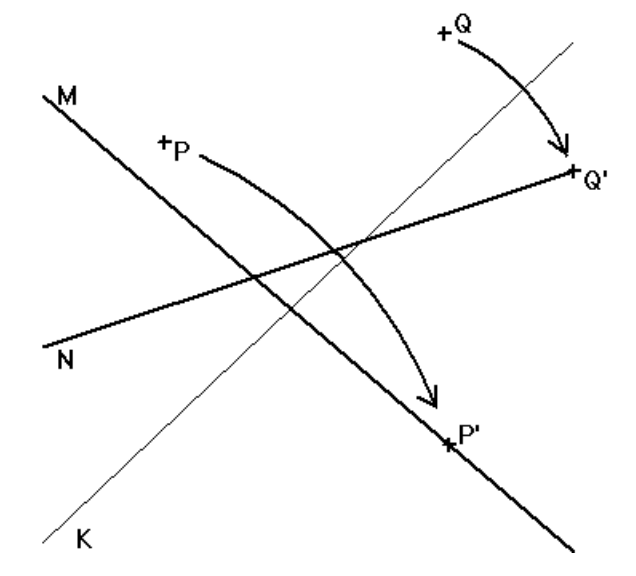시간 제한 메모리 제한 제출 정답 맞은 사람 정답 비율
1 초 512 MB 0 0 0 0.000%

## 문제

An origami program needs routines to compute where to fold the paper. The most complex fold is Beloch’s Fold, in which two points P and Q are given along with two lines M and N. The problem is to find a fold which takes P onto line M and simultaneously, takes Q onto line N. In the figure below, folding along the line K is one possible solution.Write a program to compute the fold line K, which takes point P to line M and point Q to line N, given points P and Q and lines M and N. Point P will not be on line M, point Q will not be on line N and lines M and N will not be parallel.

## 입력

nput consists of a single line of input. The line contains 10 space separated floating point values between -10000 and 10000: Px, Py, Ma, Mb, Mc, Qx, Qy, Na, Nb, Nc where:

• P = (Px, Py)
• Q = (Qx ,Qy)
• The equation of line M is: Ma*x + Mb*y + Mc = 0
• The equation of line N is: Na*x + Nb*y + Nc = 0.

## 출력

The output consists of a single line containing 3 space separated floating point values to 4 decimal places: Ka, Kb, Kc where:

Ka*x + Kb*y + Kc = 0 is a fold line which takes P onto M and Q onto N.

## 예제 입력 1

-4 5 6 7 8 9 10 -1 3 4


## 예제 출력 1

1.7691 1.1979 3.3690


## 예제 입력 2

4 5 6 7 8 9 10 -3 2 1


## 예제 출력 2

4.4949 5.7186 -20.1193


## 노트

1. Any non-zero multiple of a line equation is an equation for the same line
2. There may be more than one valid fold line. The validator will test whether folding at your fold line takes point P to line M and point Q to line N.